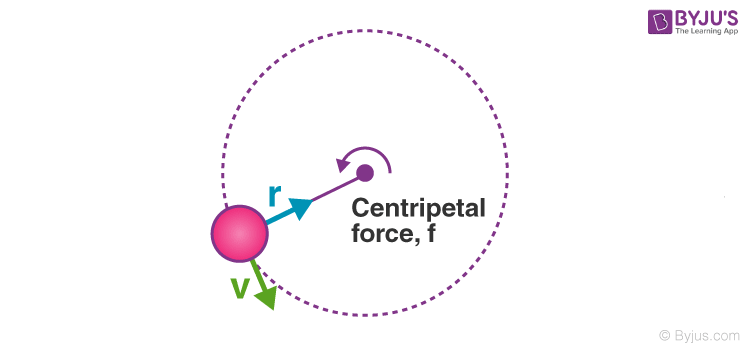Checkout JEE MAINS 2022 Question Paper Analysis : Checkout JEE MAINS 2022 Question Paper Analysis :

# Centripetal Acceleration

## What Is Centripetal Acceleration?

Centripetal acceleration is defined as the property of the motion of an object, traversing a circular path. Any object that is moving in a circle and has an acceleration vector pointed towards the center of that circle is known as Centripetal acceleration. You must have seen various examples of centripetal acceleration in your everyday life. If you drive a car around a circle then your car is undergoing centripetal acceleration and a satellite orbiting the earth also follows a centripetal acceleration. Centripetal means towards the center.## What Is Centripetal Force?

The acceleration that is directed radially towards the center of the circle having a magnitude equal to the square of the speed of the body along the curve is divided by the total distance from the center of the circle to the moving body. The force which causes the acceleration is directed towards the center of the circle and is called a centripetal force.

## Centripetal Acceleration Formula

Acceleration can be measured in meters per second as it is the number of meters per second by which your velocity changes every second. When an object is moving in a circular motion, it can be measured by using the following equation-

 $$\begin{array}{l}a_{c}=\frac{v^{2}}{r}\end{array}$$

Where,

• ac is the centripetal acceleration in m.s-2
• v is the velocity in m.s-1
• r is the radius in m

Acceleration is the change in velocity. There must be a question arising in your mind that how can something that is moving at a constant speed in a circle have an acceleration? Speed is how fast an object is moving and is scalar as it does not have any direction. Velocity is both the speed and direction and is a vector as it does not have a direction. For example – Speed is  5 miles per hour and Velocity is 5 miles per hour south.

A force always causes the centripetal acceleration as for a satellite, it is the force of gravity. For a swing-ball, it is the tension in the string. For a moving car around a car, it is the frictional force between the car and the road. If you tend to remove the centripetal acceleration then the object will continue to move in a straight line(tangent to the circle).

Q1. Name the force responsible for bending the straight path of an object to a circular path.
Ans: Centripetal force is responsible for bending the straight path of an object to a circular path.

Q2. What is the similarity between centripetal force and centripetal acceleration?
Ans: The similarity between centripetal force and centripetal acceleration is that they have the same direction.

Q3. What is the unit of centripetal acceleration?
Ans: The unit of centripetal acceleration is m.s-2.

Q4. What is the dimensional formula of centripetal acceleration?
Ans: The dimensional formula of centripetal acceleration is given as:
Dimensional formula of velocity = M0L1T-1
Dimensional formula of radius = M0L1T0
Substituting the above in the equation of centripetal acceleration formula, we get:
Dimensional formula of centripetal acceleration = M0L1T-2

Related Physics Articles:

From the video learn the concept of centripetal acceleration in detail.